# Top 10 home loan offset calculators reviewed – are they correct?

### How does home loan offset work?

An offset account is a transaction account that can be linked to your home or investment loan. The credit balance of your transaction account is offset daily against your outstanding loan balance, reducing the interest payable on that loan. With a 100% offset account the balance of the offset account is taken away from the principal remaining on the loan for interest calculation.

Let’s have a look at an example. A customer with a \$300,000 home loan over 25 years had an offset account linked to the home loan for the entire loan term with a constant balance of \$50,000 in it, they would only pay interest on \$250,000 for the entire loan term.

### How much you can save?

So exactly how much you can save with an offset account? It depends on several things:

• Loan Amount
• Loan Term
• Loan Interest
• Loan Repayment Frequency
• Offset Account Balance

For most of us in order to estimate how much we can save, we need a calculator. Most of the lenders now provide an online mortgage offset calculator that we can use.

I think it is important to understand if those online offset calculators are accurate based on the assumptions they used. In the next section I will share the results from the top 10 home loan offset calculators suggested by Google if you search “home loan offset calculator” through it.

### Top 10 home loan offset calculators

This screenshot below showed the top 10 offset calculators that had been reviewed. They includes online calculators from Westpac, ING Direct, ANZ, SunCorp, AFG (Australian Finance Group), Finder.com.au, CUA, AMP, Bankwest, and SmartSearchFinance.com.au. You can find the screenshots of all these 10 calculators at the end of this article.### The results from the top 10 offset calculators

When I reviewed the top 10 home loan offset calculators, I used the following assumptions:

• Loan Amount: \$300,000
• Loan Term: 25 years
• Loan Interest: 6.00% per annum
• Loan Repayment Frequency: monthly which means you make 12 repayments
• Loan Type: principal and interest
• Offset Account Balance: \$50,000

The table below shows the results from the top 10 home loan offset calculators: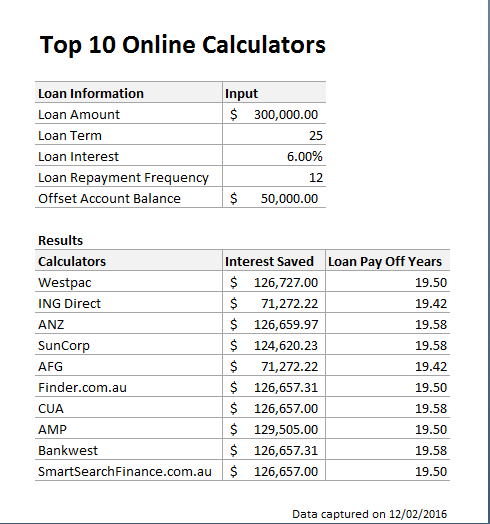As one can see, most of the calculators estimated that you would save around \$126,500 to \$126,700 over the entire loan term. AMP’s estimation is the highest at \$129,505 while ING Direct and AFG estimated the savings were only about \$71,272. In terms of loan pay-off time, the 10 calculators all estimated around 19 years and 6 months with 1 month variation. Hence, it seems that there is no issue with the pay off time.

However, the savings estimated by ING Direct and AFG seem way off from the others. Let’s see if they are wrong.

### The right savings amount

In order to work our the savings from an offset account, we need to build a calculator in Excel  to compare with and without offset account. Let’s see an example.

At the end the first month, you will need to pay the scheduled Monthly Repayment of \$1,932.90. This is calculated by using Excel PMT function:

Monthly Repayment=PMT(Loan Interest/12, Loan Term*12,-Loan Amount)=PMT(6%/12, 25*12, -300,000)=\$1,932.90

Out of this \$1,932.90 repayment,  \$432.90 is the principal payment and it is calculated by using Excel PPMT function:

PPMT(Loan Interest/12,1,Loan Term*12,-Loan Amount)=PPMT(6%/12,1,25*12,-300,000)=\$432.90

However, since there is an offset account, the interest you pay will be reduced by a amount calculated using this formula:

Offset Account Balance*Loan Interest/12=50,000*6%/12=\$250.

In other words, this \$250 will become principal repayment as you don’t need to pay this \$250 loan interest. Hence at the end of 1st month you actually pay back \$682.90 principal instead of the scheduled \$432.90.

If we continue this calculation process, we will be able to find our when the loan will be paid off and how much we can save on interests.

I have built a simple home loan offset calculator in Excel based on the calculation process described above. You can download the calculator here. Below is the result from this free calculator.Based on the calculator I built, you can save \$126,657.31 interests and the loan will be paid off at the end of 19 years 7 months.

It suggests that the online calculators from the following lenders are more accurate:

• Finder.com.au
• CUA
• Bankwest
• SmartSearchFinance.com.au

The worst online home loan offset calculators are those from:

• ING Direct
• AFG (Australian Finance Group)

### Screenshots of the top 10 online home loan offset calculators

Westpac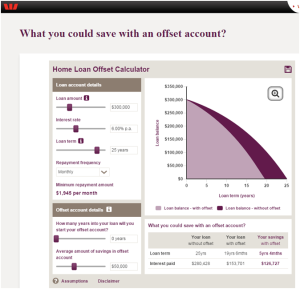ING Direct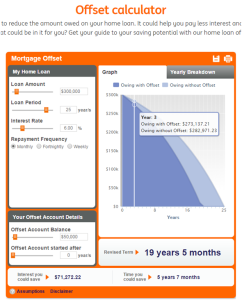ANZ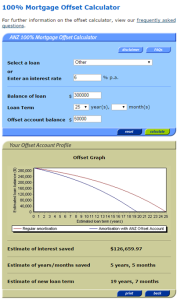SunCorp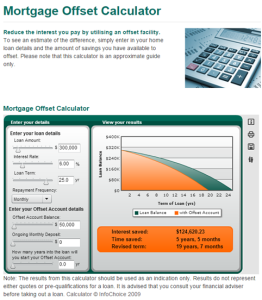AFG (Australian Finance Group)Finder.com.au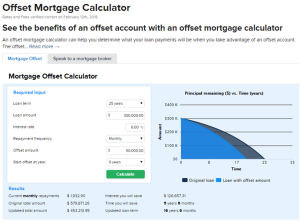CUA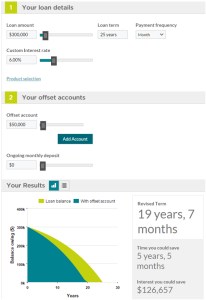AMPBankwest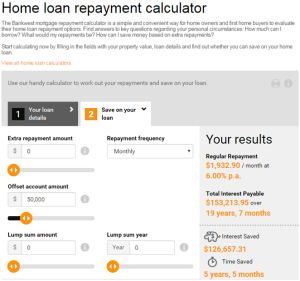SmartSearchFinance.com.au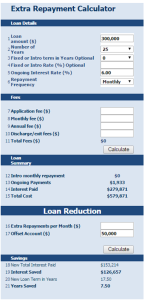## 4 Replies to “Top 10 home loan offset calculators reviewed – are they correct?”

1. Kang says:

Don’t agree. Different off-set calculators are based on different assumptions. Your formula is just based on one of these assumptions.

If you can write various formula to replicate each offset calculator’s results, you may understand the backend assumptions are different.

We can discus via my email.

1. Hi Kang,

The ING calculator is based on the following assumptions:

Calculator Assumptions

Length of Month
All months are assumed to be of equal length. In reality, many loans accrue interest on a daily basis which means monthly interest charges may vary depending on the number of days in the particular month.

Number of Weeks & Fortnights in a Year
One year is assumed to contain exactly 52 weeks or 26 fortnights. This implicitly assumes that a year has 364 days rather than the actual 365 or 366.

Rounding of Amount of Each Repayment
In practice, repayments are rounded to at least the nearer cent. However the calculator uses the unrounded repayment to derive the amount of interest payable at points along the graph and in total over the full term of the loan. This assumption allows for a smooth graph and equal repayment amounts.

Interest Rate
The interest rate used in this calculation is the ING DIRECT Orange Advantage Rate (which is a variable interest rate) and may change at any time without notice.

I don’t think they are different from the other calculators from other banks. Unless ING is not doing 100% offset, I cannot see any reason but believe the calculation behind their calculator is wrong.

I think I can duplicate their results and show you what is wrong.

Cheers,

Patrick

1. Kang says:

We are the developer of some above calculators. We can give the formula to replicate all results of all above calculators.

In formula and calculation, two main assumptions make the difference:

1) assumptions on compounding: one is based daily 30 days in a month, the other one is “same as deposit frequency”. ANZ uses the first, others use second for simplicity.
2) Definition on “Saving Amount”. If you believe all interest from offset account must be included in “Saving Amount”, you will believe the ING and AFG are wrong.
However if you have no loan, but have a saving account. If you earn interest, this interest is your income, not a svaing from any loan.

There are two formula here: A) Saving time X number of regular repayments. This is used by most calculators (Others)
B) Calculate interest difference between a loan with offset and a loan without offset (ING and AFG)
The AFG and ING has a correct logic, an offset loan is equivalent to extra repayment loan, where the extra repayment is equal to interest earned from offset account.

Why finance people do love other results which are much higher? The reason is good for marketing.
But we should not include part of our income as part of “Saving Amount” from a loan.

I think it better to compare formula and assumptions.

If you have a Saving account to earn interest, you need to pay tax. However if you arrange an offset loan and use this interest to offset a loan, we can ask ATO if you don’t need to tax any more?
This is why ING and AFG are correct in this sense.

Hope this explain what happen in these calculators

Kang

1. Hi Kang,

1) assumptions on compounding: one is based daily 30 days in a month, the other one is “same as deposit frequency”. ANZ uses the first, others use second for simplicity.

I understand this and that’s why I chose monthly repayment. This removes the difference between the two methods you mentioned. BTW I think you mean “same as loan repayment frequency”?

2) Definition on “Saving Amount”. If you believe all interest from offset account must be included in “Saving Amount”, you will believe the ING and AFG are wrong.

You misunderstood how offset works. Instead of receiving interest on the linked offset account, the interest on the loan is calculated only on the net balance of the loan balance less the amount in the linked offset account. The regular/scheduled repayment is calculated on the full amount of the loan, however, so making regular repayments will pay off the loan faster than a standard loan without offset.

So offset is not “equivalent to extra repayment loan, where the extra repayment is equal to interest earned from offset account” as you don’t earn any interest from the linked account and you don’t make any extra repayment (though the effect is as same as you earn interest and make extra repayment) – you simply pay less interest because offset reduces your loan balance.

There are two formula here: B) Calculate interest difference between a loan with offset and a loan without offset (ING and AFG)

This is exactly what other banks showed and where the results from ING and AFG failed to show.

If you have a Saving account to earn interest, you need to pay tax.

This is correct. However like I said before you don’t earn interest from the linked account so this is no income. Since this is no income, you don’t pay any tax. You can check with your accountant on this.

In essence there is no money moved from the linked offset account to your loan account. Your understanding is that you move money (interest earned) from the linked account to your loan account while this is not true as you don’t earn interest on the linked offset account. The difference can be illustrated by the example below:

Loan balance \$300,000
Interest 6%
Loan term 25 years
Offset balance \$50,000

So for the first month based on your logic/understanding it will be:

The \$50,000 earns you \$250 interest (\$50,000×6%/12=\$250) for the month. The bank deposits this \$250 into the linked offset account and you (or the bank) then move this \$250 to your loan account so to make extra repayment. You treat the \$250 as an income so the interest saved for the first month will be \$0.

The correct logic should be:

You pay this amount of interest (\$300,000 – \$50,000)*6%/12 = \$1250 for the first month. Out of the \$1,932.90 scheduled monthly repayment the scheduled interest should be \$1,500.00. Since you only need to pay \$1,250 interest, effectively you reduce your loan balance by \$1,500 – \$1,250=\$250. The interest saved for the first month is \$250.